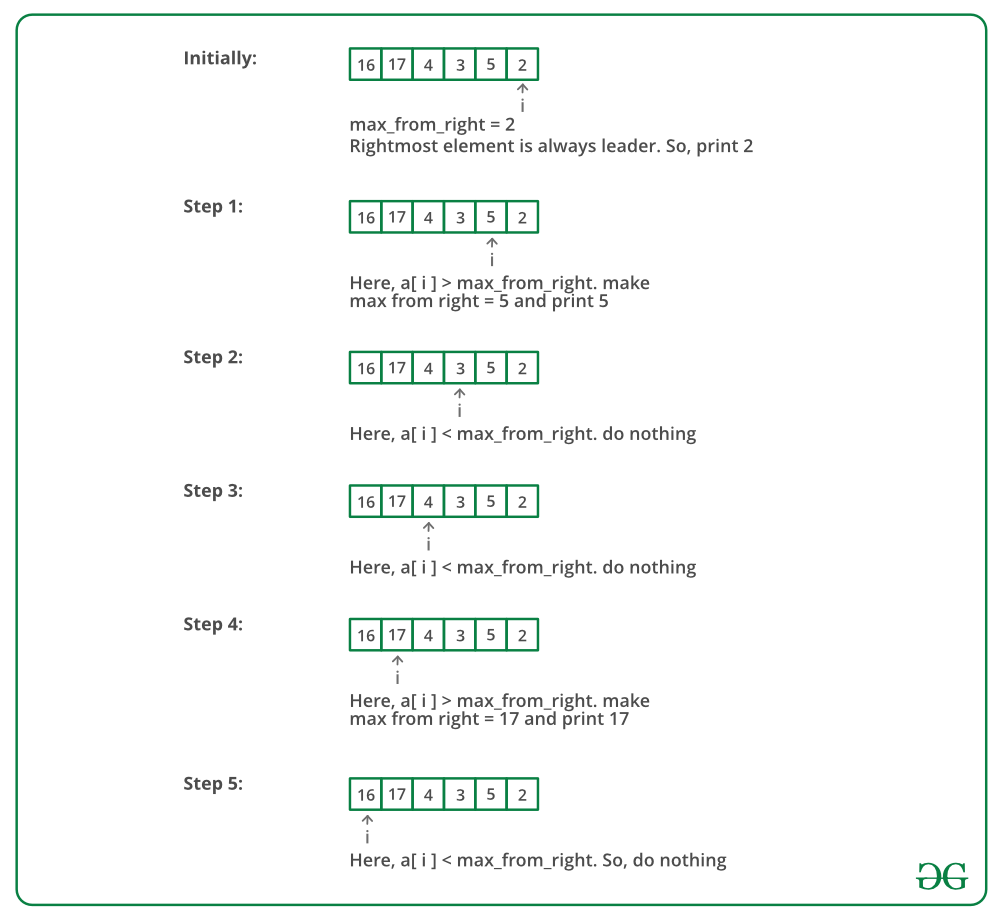• Difficulty Level : Easy
• Last Updated : 11 Jun, 2022

Write a program to print all the LEADERS in the array. An element is leader if it is greater than all the elements to its right side. And the rightmost element is always a leader. For example in the array {16, 17, 4, 3, 5, 2}, leaders are 17, 5 and 2.
Let the input array be arr[] and size of the array be size.

Method 1 (Simple)
Use two loops. The outer loop runs from 0 to size – 1 and one by one picks all elements from left to right. The inner loop compares the picked element to all the elements to its right side. If the picked element is greater than all the elements to its right side, then the picked element is the leader.

## C++

 `#include``using` `namespace` `std;` `/*C++ Function to print leaders in an array */``void` `printLeaders(``int` `arr[], ``int` `size)``{``    ``for` `(``int` `i = 0; i < size; i++)``    ``{``        ``int` `j;``        ``for` `(j = i+1; j < size; j++)``        ``{``            ``if` `(arr[i] <=arr[j])``                ``break``;``        ``}   ``        ``if` `(j == size) ``// the loop didn't break``            ``cout << arr[i] << ``" "``;``  ``}``}` `/* Driver program to test above function */``int` `main()``{``    ``int` `arr[] = {16, 17, 4, 3, 5, 2};``    ``int` `n = ``sizeof``(arr)/``sizeof``(arr);``    ``printLeaders(arr, n);``    ``return` `0;``}`

## Java

 `class` `LeadersInArray``{``    ``/*Java Function to print leaders in an array */``    ``void` `printLeaders(``int` `arr[], ``int` `size)``    ``{``        ``for` `(``int` `i = ``0``; i < size; i++)``        ``{``            ``int` `j;``            ``for` `(j = i + ``1``; j < size; j++)``            ``{``                ``if` `(arr[i] <=arr[j])``                    ``break``;``            ``}``            ``if` `(j == size) ``// the loop didn't break``                ``System.out.print(arr[i] + ``" "``);``        ``}``    ``}` `    ``/* Driver program to test above functions */``    ``public` `static` `void` `main(String[] args)``    ``{``        ``LeadersInArray lead = ``new` `LeadersInArray();``        ``int` `arr[] = ``new` `int``[]{``16``, ``17``, ``4``, ``3``, ``5``, ``2``};``        ``int` `n = arr.length;``        ``lead.printLeaders(arr, n);``    ``}``}`

## Python3

 `# Python Function to print leaders in array` `def` `printLeaders(arr,size):``    ` `    ``for` `i ``in` `range``(``0``, size):``        ``for` `j ``in` `range``(i``+``1``, size):``            ``if` `arr[i]<``=``arr[j]:``                ``break``        ``if` `j ``=``=` `size``-``1``: ``# If loop didn't break``            ``print` `(arr[i],end``=``' '``)` `# Driver function``arr``=``[``16``, ``17``, ``4``, ``3``, ``5``, ``2``]``printLeaders(arr, ``len``(arr))` `# This code is contributed by _Devesh Agrawal__`

## C#

 `// C# program to print``// leaders in array``using` `System;``class` `GFG``{``    ``void` `printLeaders(``int` `[]arr,``                      ``int` `size)``    ``{``        ``for` `(``int` `i = 0; i < size; i++)``        ``{``            ``int` `j;``            ``for` `(j = i + 1; j < size; j++)``            ``{``                ``if` `(arr[i] <=arr[j])``                    ``break``;``            ``}``            ` `            ``// the loop didn't break``            ``if` `(j == size)``                ``Console.Write(arr[i] + ``" "``);``        ``}``    ``}` `    ``// Driver Code``    ``public` `static` `void` `Main()``    ``{``        ``GFG lead = ``new` `GFG();``        ``int` `[]arr = ``new` `int``[]{16, 17, 4, 3, 5, 2};``        ``int` `n = arr.Length;``        ``lead.printLeaders(arr, n);``    ``}``}` `// This code is contributed by``// Akanksha Rai(Abby_akku)`

## PHP

 ``

## Javascript

 ``

Output

`17 5 2 `

Time Complexity: O(n*n)

Auxiliary Space: O(1)
Method 2 (Scan from right)
Scan all the elements from right to left in an array and keep track of maximum till now. When maximum changes its value, print it.
Below image is a dry run of the above approach:Below is the implementation of the above approach:

## C++

 `#include ``using` `namespace` `std;` `/* C++ Function to print leaders in an array */``void` `printLeaders(``int` `arr[], ``int` `size)``{``    ``int` `max_from_right =  arr[size-1];` `    ``/* Rightmost element is always leader */``    ``cout << max_from_right << ``" "``;``    ` `    ``for` `(``int` `i = size-2; i >= 0; i--)``    ``{``        ``if` `(max_from_right < arr[i])``        ``{          ``            ``max_from_right = arr[i];``            ``cout << max_from_right << ``" "``;``        ``}``    ``}   ``}` `/* Driver program to test above function*/``int` `main()``{``    ``int` `arr[] = {16, 17, 4, 3, 5, 2};``    ``int` `n = ``sizeof``(arr)/``sizeof``(arr);``    ``printLeaders(arr, n);``    ``return` `0;``}   `

## Java

 `class` `LeadersInArray``{``    ``/* Java Function to print leaders in an array */``    ``void` `printLeaders(``int` `arr[], ``int` `size)``    ``{``        ``int` `max_from_right =  arr[size-``1``];`` ` `        ``/* Rightmost element is always leader */``        ``System.out.print(max_from_right + ``" "``);``     ` `        ``for` `(``int` `i = size-``2``; i >= ``0``; i--)``        ``{``            ``if` `(max_from_right < arr[i])``            ``{          ``            ``max_from_right = arr[i];``            ``System.out.print(max_from_right + ``" "``);``            ``}``        ``}   ``    ``}` `    ``/* Driver program to test above functions */``    ``public` `static` `void` `main(String[] args)``    ``{``        ``LeadersInArray lead = ``new` `LeadersInArray();``        ``int` `arr[] = ``new` `int``[]{``16``, ``17``, ``4``, ``3``, ``5``, ``2``};``        ``int` `n = arr.length;``        ``lead.printLeaders(arr, n);``    ``}``}`

## Python3

 `# Python function to print leaders in array``def` `printLeaders(arr, size):``   ` `    ``max_from_right ``=` `arr[size``-``1``]  ``    ``print` `(max_from_right,end``=``' '``)   ``    ``for` `i ``in` `range``( size``-``2``, ``-``1``, ``-``1``):       ``        ``if` `max_from_right < arr[i]:       ``            ``print` `(arr[i],end``=``' '``)``            ``max_from_right ``=` `arr[i]``        ` `# Driver function``arr ``=` `[``16``, ``17``, ``4``, ``3``, ``5``, ``2``]``printLeaders(arr, ``len``(arr))` `# This code contributed by _Devesh Agrawal__`

## C#

 `// C# program to find Leaders in an array``using` `System;` `class` `LeadersInArray {``    ` `    ``// C# Function to print leaders``    ``// in an array``    ``void` `printLeaders(``int` `[]arr, ``int` `size)``    ``{``        ``int` `max_from_right = arr[size - 1];` `        ``// Rightmost element is always leader``        ``Console.Write(max_from_right +``" "``);``    ` `        ``for` `(``int` `i = size - 2; i >= 0; i--)``        ``{``            ``if` `(max_from_right < arr[i])   ``            ``{    ``                ``max_from_right = arr[i];``                ``Console.Write(max_from_right +``" "``);``            ``}``        ``}``    ``}` `    ``// Driver Code``    ``public` `static` `void` `Main(String[] args)``    ``{``        ``LeadersInArray lead = ``new` `LeadersInArray();``        ``int` `[]arr = ``new` `int``[]{16, 17, 4, 3, 5, 2};``        ``int` `n = arr.Length;``        ``lead.printLeaders(arr, n);``    ``}``}` `// This code is contributed``// by Akanksha Rai(Abby_akku)`

## PHP

 `= 0; ``\$i``--)``    ``{``        ``if` `(``\$max_from_right` `< ``\$arr``[``\$i``])   ``        ``{        ``            ``\$max_from_right` `= ``\$arr``[``\$i``];``            ``echo``(``\$max_from_right``);``            ``echo``(``" "``);``        ``}``    ``}``}` `// Driver Code``\$arr` `= ``array``(16, 17, 4, 3, 5, 2);``\$n` `= sizeof(``\$arr``);``printLeaders(``\$arr``, ``\$n``);` `// This code is contributed``// by Shivi_Aggarwal``?>`

## Javascript

 ``

Output

`2 5 17 `

Time Complexity: O(n)
Auxiliary Space: O(1)

Method 3:

In method 2, we get time complexity O(n), but the output we get is not in the same order as the elements appear in our input array, so to get out output in the same order as in the input array, we can use stack data structure.

Below is the implementation of the above approach:

## C++

 `#include ``using` `namespace` `std;` `/* C++ Function to print leaders in an array */``void` `printLeaders(``int` `arr[], ``int` `size)``{``      ``/* create stack to store leaders*/``      ``stack<``int``> sk;``      ``sk.push(arr[size-1]);``  ` `    ``for` `(``int` `i = size-2; i >= 0; i--)``    ``{``        ``if``(arr[i] > sk.top())``        ``{         ``            ``sk.push(arr[i]);``        ``}``    ``}  ``  ` `      ``/* print stack elements*/``      ``/* run loop till stack is not empty*/``      ``while``(!sk.empty()){     ``        ``cout<

Output

`17 5 2 `

Time complexity: O(n)

Auxiliary space: O(n)